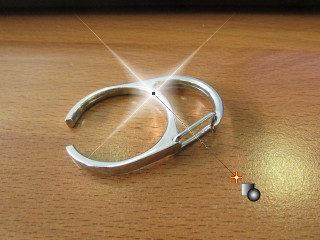...GT Shape Tool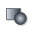Draws a shape in which the color gradually changes from [color #1 + alpha1] to [color #2 + alpha2]. Hold the Shift button to constrain it to a regular shape (e.g. square or circle). Where applicable, this tool responds to the width and level settings. The size of the area can be controlled using the snap-to-grid feature, in which case the tool will correct the box size to always be a multiple of the grid size.

 OptionsRectangle, Horizontal This option draws a simple rectangle filled with a horizontal gradient.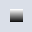Rectangle, Vertical This option draws a simple rectangle filled with a vertical gradient.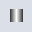Double Rectangle, Horizontal This option draws a simple rectangle filled with a reflected horizontal gradient.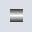Double Rectangle, Vertical This option draws a simple rectangle filled with a reflected vertical gradient.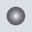Ellipse This option draws an ellipsefilled with an elliptical gradient.Angular Rect This option draws a rectangle at angle. The rectangle is filled with a gradient in the direction of its minor axis.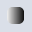Rounded Rectangle, Horizontal This option draws a rectangle with rounded edges. The rectangle is filled with a horizontal gradient.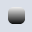Rounded Rectangle, Vertical This option draws a rectangle with rounded edges. The rectangle is filled with a vertical gradient.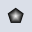Polygon This option draws an n-sided polygon. The number of sides is controlled by the level parameter; for example, it draws a pentagon if level=5 or an octagon if level=8. The polygon is filled with a gradient of the same shape radiating from the center.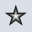Star Polygon This option draws an n-pointed star. The number of points is controlled by the level parameter; for example, it draws a five-pointed star if level=5 or an eight-pointed star if level=8. The star is filled with a gradient of the same shape radiating from the center.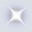Sparkle This option draws a sparkle - a 'bling-bling' effect, so to speak. Very useful in painting shiny objects, and yes, that includes gold chains and spinners.